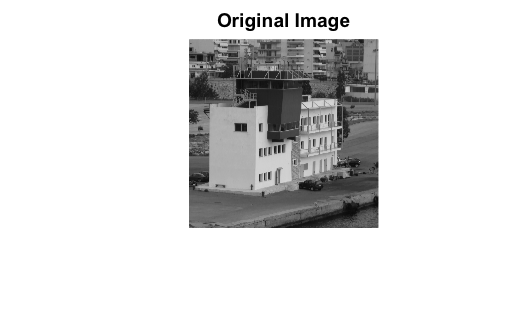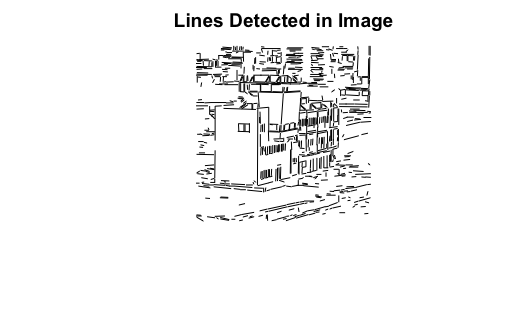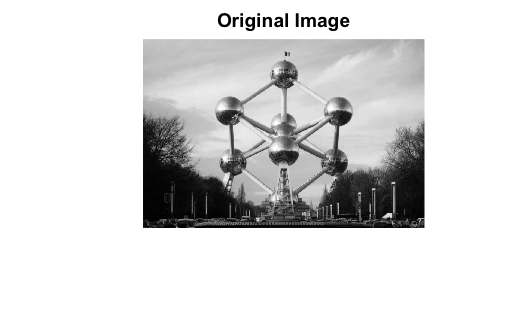Read in a pgm image image with values in the 0-255 range.

``````library(image.LineSegmentDetector)
library(pixmap)
image <- read.pnm(file = system.file("extdata", "le-piree.pgm",
package = "image.LineSegmentDetector"),
cellres = 1)``````

## Plot Detected Lines

``plot(image, main = "Original Image")````````linesegments <- image_line_segment_detector(image@grey * 255)
plot(linesegments, main = "Lines Detected in Image")``````## Convert Image to pgm

If you have another type of image (jpg, png, …). Convert the image to pgm format before getting the 0-255 range or convert it to grey format before passing it on to the function.

``````library(magick)
f <- tempfile(fileext = ".pgm")
"atomium.jpg",
package = "image.LineSegmentDetector"))
# conver image to pgm
x <- image_convert(x, format = "pgm", depth = 8)
image_write(x, path = f, format = "pgm")``````

## Plot Lines Over Image

``````# load pgm image into memory
image <- read.pnm(file = f, cellres = 1)
# create line segments from pgm image
linesegments <- image_line_segment_detector(image@grey * 255)
# plot detected lines
plot(image, main = "Original Image")````````````plot(image, main = "Image with Detected Lines in Red")
plot(linesegments, add = TRUE, col = "red")``````